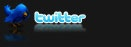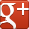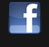top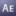# Exponential Scale live with expressions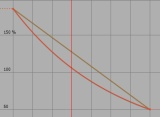Once you run the animation assistant for Exponential Scale, the keyframes are locked down and if you need to change the start or end values, you have to delete all the intermediate keframes and re-run the animation assistant. Here is a way to replicate the Exponential Scale via expression.
After Effects CS3
Useful things
Expressions

Views: 11661
"For two keyframes it should give the same result as if you applied AE's exponential scale. If there are more than two keyframes, it will apply the exponential scale between each adjacent pair of keyframes. Should work for multi-dimensional properties as well." (from Dan Ebberts via Adobe Forums)

Just Alt-Click on the Scale Stopwatch and paste this expression:
``````//applyTo: scale
n = 0;
if (numKeys > 0){
n = nearestKey(time).index;
if (key(n).time > time){
n--;
}
}

if (n == 0 || n == numKeys){
value
}else{
d = 1
try{
value;
d++;
value;
d++;
value;
d++;
}catch (err){
}
t1 = key(n).time;
t2 = key(n+1).time;
if (d == 1){
v1 = key(n).value;
if (v1 == 0) v1 = .0001;
v2 = key(n+1).value;
if (v2 == 0) v2 = .0001;
k = Math.log(v1/v2)/(t2-t1);
v1/Math.exp((time-t1)*k)
}else{
v = [];
for(i = 0; i < d; i++){
v1 = key(n).value[i];
if (v1 == 0) v1 = .0001;
v2 = key(n+1).value[i];
if (v2 == 0) v2 = .0001;
k = Math.log(v1/v2)/(t2-t1);
v[i] = v1/Math.exp((time-t1)*k)
}
v
}
}``````
Navarro Parker has made the preview testmovie

### Keywords

Exponential Scale

(Min. Version: After Effects CS3, Category: Useful things, Type: Expressions)
posted by vfx007 on Aug 25, 2009

## Related topics:# Auto Layer Flip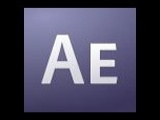Apply this expression to your 'Scale' attribute and it'll automatically 'flip' the layer in 3d space when the camera goes behind it. This way, your text will never be seen from behind, for instance.
After Effects 6
Camera
Expressions

Views: 5982
posted by MicGyver on Sep 15, 2008# Scale relative to distance from cameraThis expression maintains the objects apparent size as it moves away from camera.
It's helpful for taking a flat layout and making a "multiplane" layout from it for animation.
After Effects 6
Camera
Expressions

Views: 17751
posted by Dan Johansson on Sep 15, 2008# Animate scale at each layer-time markerAfter Effects CS3
Useful things
Expressions

Views: 5578
posted by MicGyver on Sep 16, 2008